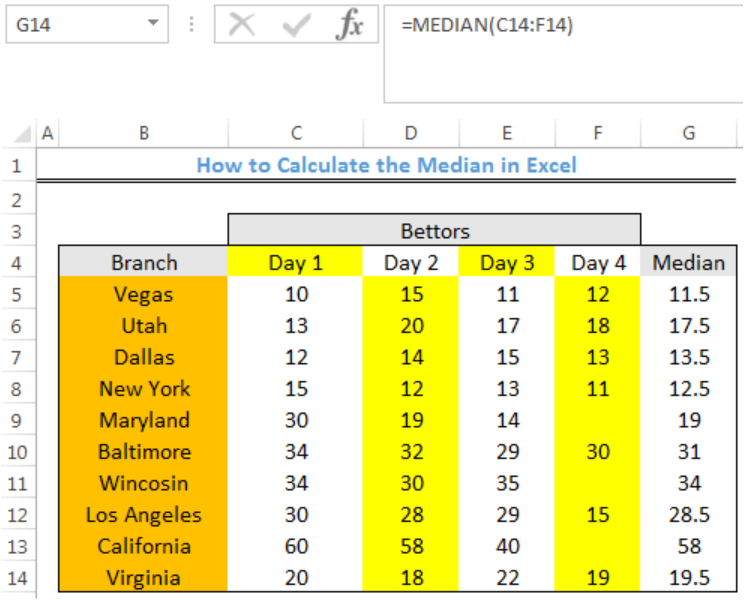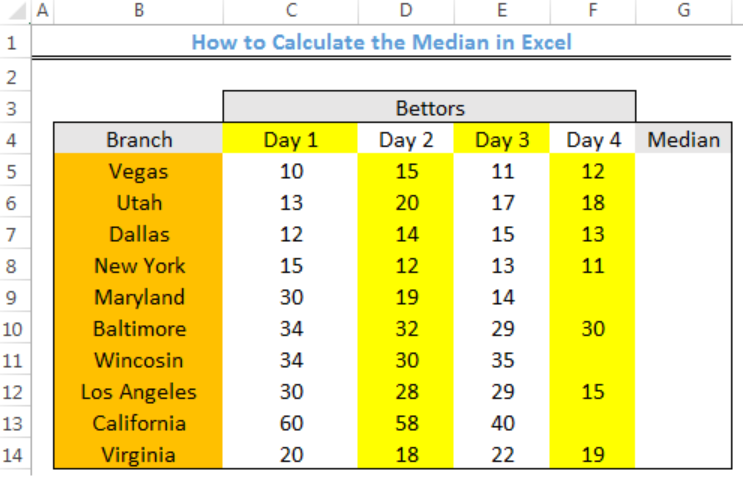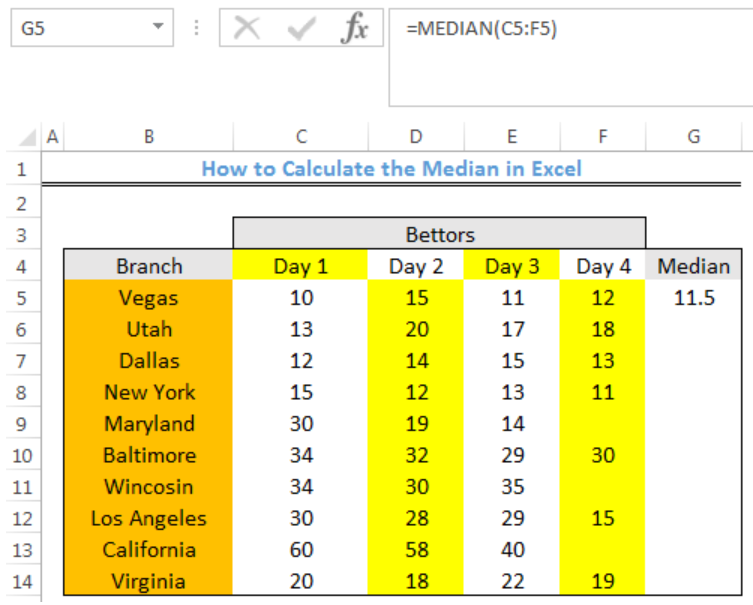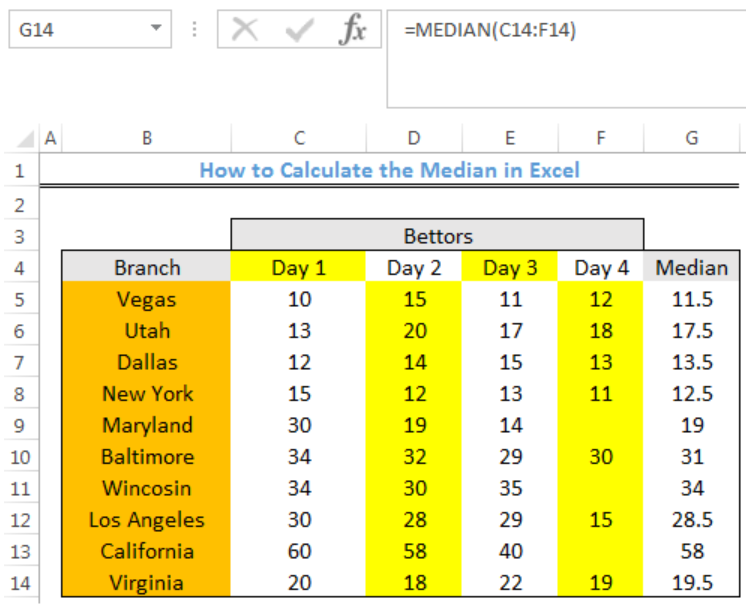Get instant live expert help with Excel or Google Sheets“My Excelchat expert helped me in less than 20 minutes, saving me what would have been 5 hours of work!”

#### Post your problem and you’ll get expert help in seconds.

Your message must be at least 40 characters
Our professional experts are available now. Your privacy is guaranteed.

# Discover How to Calculate the Median in Excel

We can use the MEDIAN function to return the middle number in a range. If the total numbers in the range are odd, the middle number is taken as the median. If the total numbers in the range are even, the average of the two middle numbers is taken as the median. The steps below will walk through the process.Figure 1- Final result of the MEDIAN function

## Syntax

`=MEDIAN(number1, [number2], ...)`

• number1: This is a number or cell reference that refers to numeric values
• number2 [optional]: This is a number or cell reference that refers to numeric values

## Formula

`=MEDIAN(C5:F5)`

## Setting up the Data

• The Branches of a betting store will be entered into Column B
• Column C, D, E, and F will contain the NUMBER OF BETTORS for each of the stores for 4 days
• The median for each branch will be returned in Column GFigure 2 – Setting up the Data

## Calculate the MEDIAN for each Branch

• We will click on Cell G5
• We will insert the formula below into Cell G5
`=MEDIAN(C5:F5)`
• We will press the enter keyFigure 3- Median for Vegas

• We will click on Cell G5 again
• We will double click on the fill handle tool which is the small plus sign you see at the bottom right of Cell G5. Select and drag down to copy the formula to other cells.Figure 4- Median for the Stores

## Note

• Any empty cell and cell with text or logical values within the range is ignored by the MEDIAN Function
• The numbers could be supplied to the MEDIAN function as numbers, ranges, named ranges, or cell references that contain numeric values. Up to 255 numbers can be supplied.

## Instant Connection to an Expert through our Excelchat Service

Most of the time, the problem you will need to solve will be more complex than a simple application of a formula or function. If you want to save hours of research and frustration, try our live Excelchat service! Our Excel Experts are available 24/7 to answer any Excel question you may have. We guarantee a connection within 30 seconds and a customized solution within 20 minutes.

Are you still looking for help with the Average function? View our comprehensive round-up of Average function tutorials here.

Solution examplesNeed to calculate out two equally spaced points between a range of dates. Basically like the MEDIAN function between two cells that have dates, but I need to produce two equally spaced points between that range. For example. If I have the following date range (A1) 2/2/2018 (B1) 7/2/2018, then that's roughly 150 days total. I want 4 equally spaced points (Start, Start-Mid, Mid-End, End). So I'd say that's 50 day chunks spaced euqally. Which would produce 4 total dates 2/2/2018 - 3/24/2018 - 5/13/2018 - 7/2/2018.
Solved by K. J. in 20 minsi have this formula currently which works but not if I want it for every day - any ideas ?? (NETWORKDAYS.INTL(L1362,M1362,11,BH!A:A)-1)*("17:30"-"8:30")+IF(NETWORKDAYS.INTL(M1362,M1362,11,BH!A:A),MEDIAN(MOD(M1362,1),"8:30","17:30"),"17:30")-MEDIAN(NETWORKDAYS.INTL(L1362,L1362,11,BH!A:A)*MOD(L1362,1),"8:30","17:30")
Solved by A. D. in 28 mins